+

# Odds

Author: Sophia Tutorial
##### Description:

Relate probability to odds in a given situation.

(more)

Sophia’s self-paced online courses are a great way to save time and money as you earn credits eligible for transfer to many different colleges and universities.*

No credit card required

46 Sophia partners guarantee credit transfer.

299 Institutions have accepted or given pre-approval for credit transfer.

* The American Council on Education's College Credit Recommendation Service (ACE Credit®) has evaluated and recommended college credit for 33 of Sophia’s online courses. Many different colleges and universities consider ACE CREDIT recommendations in determining the applicability to their course and degree programs.

Tutorial
what's covered
This tutorial will discuss odds--odds in favor of an event or the odds against an event. Our discussion breaks down as follows:

1. Odds
2. Converting Odds to Probability

## 1. Odds

Odds are often confused with probability. However, odds are another way to express the likelihood that is different than probability. When you say that there is a 1 in 5 probability, that will be different than saying the odds in favor are 1 in 5.

### IN CONTEXT

On this spinner, there are three 1's, a 2, two 3's, and two 4's.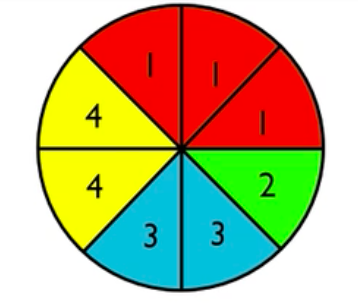Suppose that 3 is a favorable outcome. Probability is the ratio of favorable outcomes to total outcomes. Since there are two 3's on the spinner, there are two favorable outcomes out of eight total outcomes. Therefore, 1/4 is the probability of 3.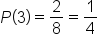Now we're going to contrast that with odds. The odds are the ratio of favorable outcomes to unfavorable outcomes. So, for a 3, there are two favorable outcomes, and there are six unfavorable outcomes. Therefore, the odds in favor of a 3 on the spinner are two to six, which can be reduced to one to three.

Odds in favor of 3 = 1:3

This says the odds in favor of a 3 are one to three, which means that for every one favorable outcome, there are three unfavorable outcomes.

Odds against are the ratio of unfavorable outcomes to the favorable outcomes, which means that there are three unfavorable to every one favorable. Odds against a 3 are three to one. Odds can also be expressed against an event simply by reversing the numbers you got when calculating the odds in favor of the event.

Odds against 3 = 3:1

Odds are usually expressed with a colon, rather than a fraction bar, although both are accepted. You should always reduce as if it was a fraction--in this case, for example, 6:2 reduced to 3:1, or 2:6 reduced to 1:3.

did you know
When odds are listed in the newspaper or a contest, typically what they're reporting are the odds against winning, such as the odds against, for example, a horse winning a particular race.

term to know

Odds
A ratio relating the number of favorable outcomes to unfavorable outcomes (odds in favor) or vice-versa (odds against). Odds are usually expressed in simplest integer terms (e.g., 2:1, not 0.5:1 or 4:2).

## 2. Converting Odds to Probability

Let's convert probability to odds by exploring a few scenarios.

Probability Odds in Favor Odds Against Explanation
P(heads on a coin) =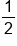1:1 1:1 On a coin, there's one favorable and one unfavorable outcome.
P(red in roulette) =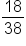18:20
=
9:10
20:18
=
10:9
On a roulette wheel, there are 18 favorable outcomes to 20 unfavorable outcomes, however, you must remember to reduce. This is also the same as 9 favorable outcomes to 10 unfavorable outcomes.
P(4 on a fair die) =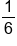1:5 5:1 On the die, there is one favorable outcome and five unfavorable outcomes.

big idea
For the odds against, you simply switch the numbers around.

Let's try another one.

try it
Determine the following odds using this jar of marbles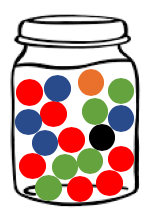Probability Odds in Favor Odds Against Explanation
P(red) =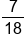7:11 11:7 Of the 18 marbles, 7 of the marbles are red, and 11 of them are not red.
P(green) =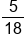5:13 13:5 Of the 18 marbles, 5 of the marbles are green, and 13 of them are not green.
P(blue) =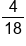=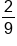4:14 = 2:7 14:4 = 7:2 Of the 18 marbles, 4 of the marbles are blue, and 14 of them are not blue. This can be reduced: for every 9 marbles, 2 are blue and 7 are not blue.
P(orange) =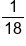1:17 17:1 Of the 18 marbles, 1 of the marbles is orange, and 17 of them are not orange.

summary
Odds are not the same as probability. The two terms should not be used interchangeably, although in real life you may hear it often. The odds in favor of an event are the favorable to unfavorable outcome ratio. The odds against an event are the unfavorable to favorable outcome ratio. Odds can be converted to probability by evaluating favorable to unfavorable outcomes.

Good luck!

Source: Adapted from Sophia tutorial by Jonathan Osters.

Terms to Know
Odds

A ratio relating the number of favorable outcomes to unfavorable outcomes (odds in favor) or vice-versa (odds against). Odds are usually expressed in simplest integer terms (eg 2:1, not 0.5:1 or 4:2).

Rating